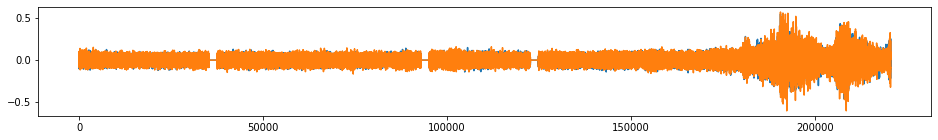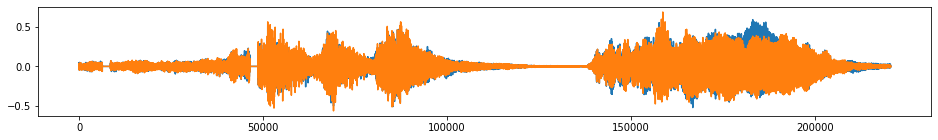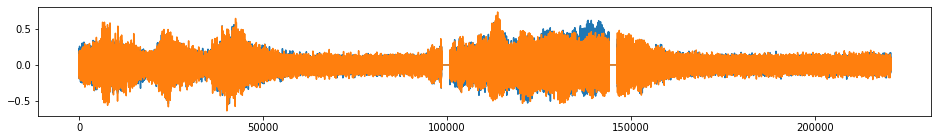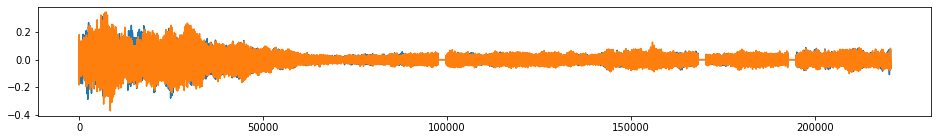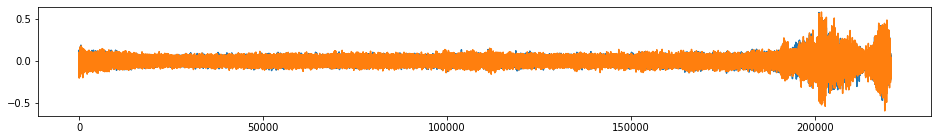# tsaug¶

tsaug is a Python package for time series augmentation. It offers a set of augmentation methods for time series, as well as a simple API to connect multiple augmenters into a pipeline.

## Installation¶

Prerequisites: Python 3.5 or later.

It is recommended to install the most recent stable release of tsaug from PyPI.

```\$ pip install tsaug
```

Alternatively, you could install from source code. This will give you the latest, but unstable, version of tsaug.

```\$ git clone https://github.com/arundo/tsaug.git
\$ cd tsaug/
\$ git checkout develop
\$ pip install ./
```

## Quick Start¶

### Augment a batch of multivariate time series¶

```>>> import numpy as np
>>> from tsaug.visualization import plot
>>> plot(X, Y)
```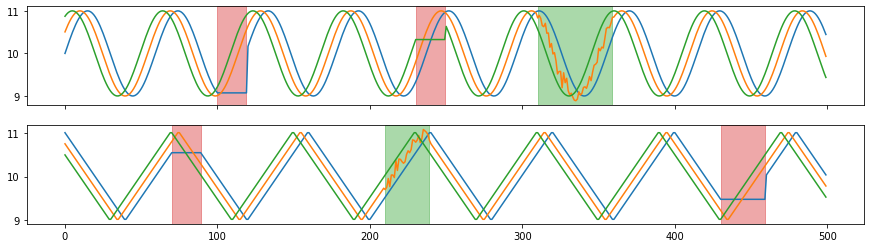1. Build a pipeline of augmenters as follows:

• random time warping 5 times in parallel,

• random crop subsequences with length 300,

• random quantize to 10-, 20-, or 30- level sets,

• with 80% probability , random drift the signal up to 10% - 50%,

• with 50% probability, reverse the sequence.

```>>> from tsaug import TimeWarp, Crop, Quantize, Drift, Reverse
>>> my_augmenter = (
...     TimeWarp() * 5  # random time warping 5 times in parallel
...     + Crop(size=300)  # random crop subsequences with length 300
...     + Quantize(n_levels=[10, 20, 30])  # random quantize to 10-, 20-, or 30- level sets
...     + Drift(max_drift=(0.1, 0.5)) @ 0.8  # with 80% probability, random drift the signal up to 10% - 50%
...     + Reverse() @ 0.5  # with 50% probability, reverse the sequence
... )
```
1. Augment the batch.

```>>> X_aug, Y_aug = my_augmenter.augment(X, Y)
>>> plot(X_aug, Y_aug)
```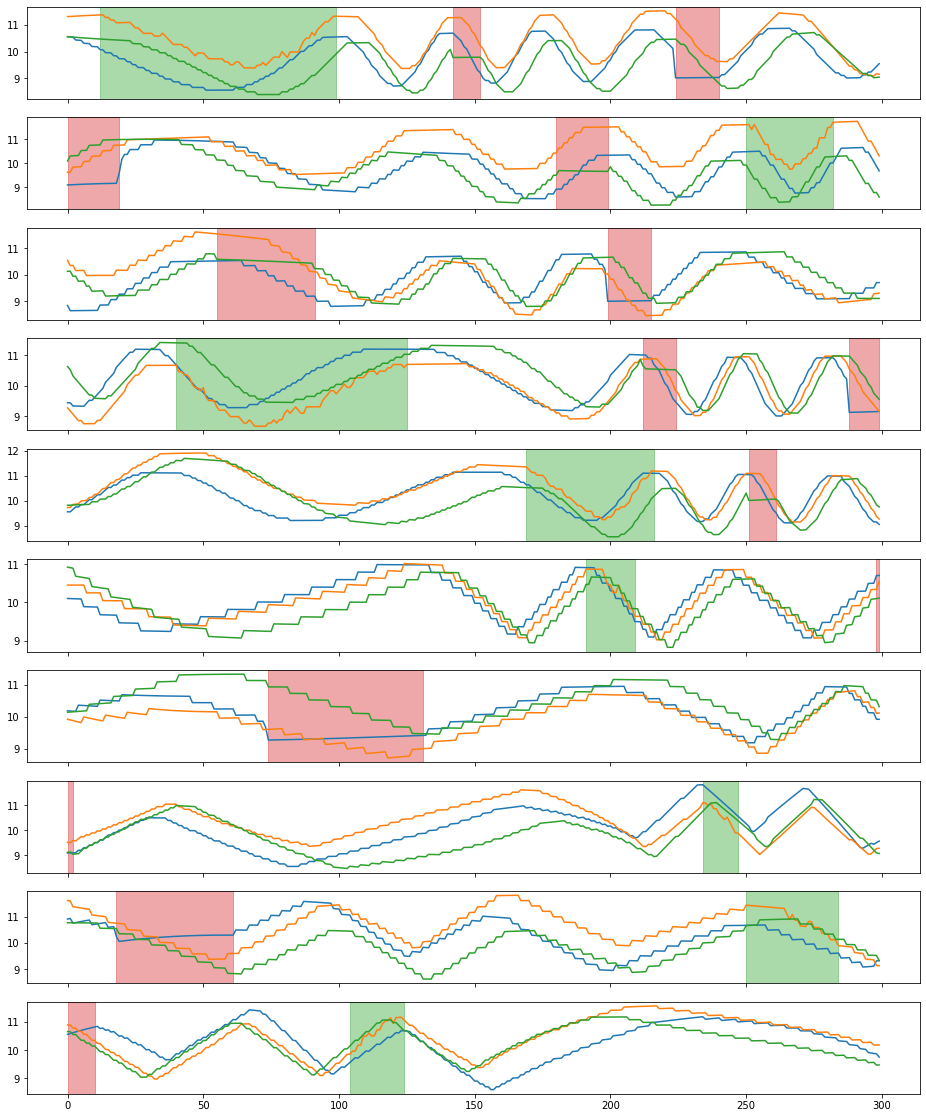### Augment a 2-channel audio sequence¶

```>>> import librosa
>>> from IPython.display import Audio
>>> beethoven, samplerate = librosa.load("./beethoven.wav", mono=False)
>>> beethoven = np.expand_dims(beethoven.swapaxes(0,1), 0) # reshape the numpy array to input of tsaug
>>> plot(beethoven)
>>> Audio(beethoven.reshape(-1, 2).T, rate=samplerate)
```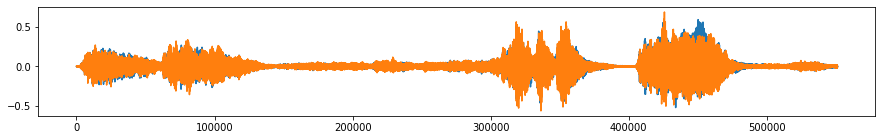1. Build a pipeline of augmenters as follows:

• random crop subsequences of 10 seconds,

• with 50% probability, add random noise up to 1% - 5%,

• drop out 10% of the time points (dropped out units are 1 ms, 10 ms, or 100 ms) and fill the dropped out points with zeros.

```>>> from tsaug import Crop, AddNoise, Dropout
>>> my_augmenter = (
...     Crop(size=samplerate * 10) * 5  # random crop subsequences of 10 seconds
...     + AddNoise(scale=(0.01, 0.05)) @ 0.5  # with 50% probability, add random noise up to 1% - 5%
...     + Dropout(
...         p=0.1,
...         fill=0,
...         size=[int(0.001 * samplerate), int(0.01 * samplerate), int(0.1 * samplerate)]
...     )  # drop out 10% of the time points (dropped out units are 1 ms, 10 ms, or 100 ms) and fill the dropped out points with zeros
... )
```
1. Augment the audio sequence.

```>>> beethoven_aug = my_augmenter.augment(beethoven)
>>> for i in range(5):
...     plot(beethoven_aug[[i]])
...     Audio(beethoven_aug[i].reshape(-1, 2).T, rate=samplerate)
```